Function Repository Resource:

# BinaryCompositions

List all possible binary compositions for a set of chosen symbols

 ResourceFunction["BinaryCompositions"][leafn,sym] returns all possible binary compositions of symbols listed in sym whose proper leaf count (terminal nodes only) equals leafn.

## Examples

### Basic Examples (3)

Axiom-level binary compositions for cases n=1,,4:

 In:=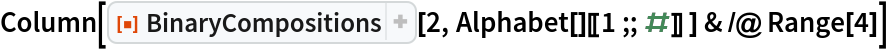Out=Double level composition acts like Tuples:

 In:=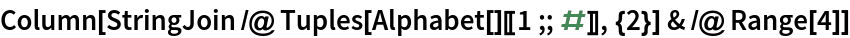Out=Binary compositions are composable:

 In:=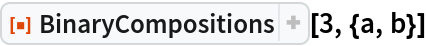Out=The recursive count of compositions relates to OEIS A025225, i.e. to the generators of 2-colored planar binary trees:

 In:=Out=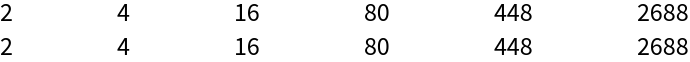The terminal node leaf count is two more than the composition depth:

 In:=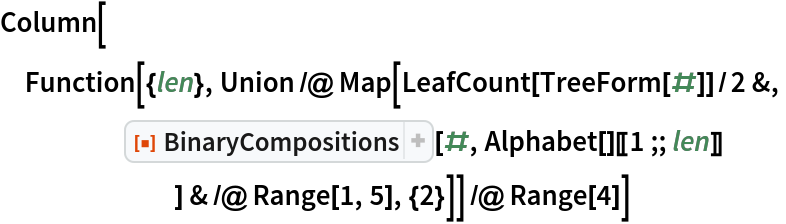Out=